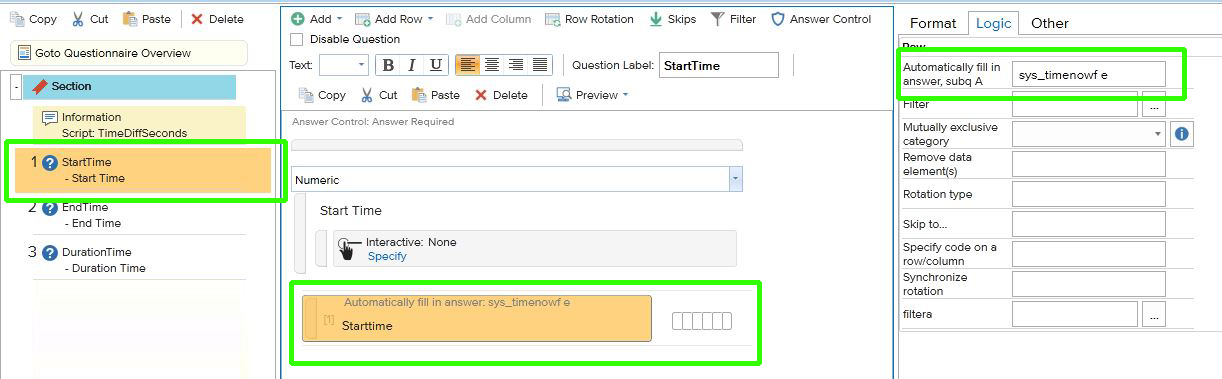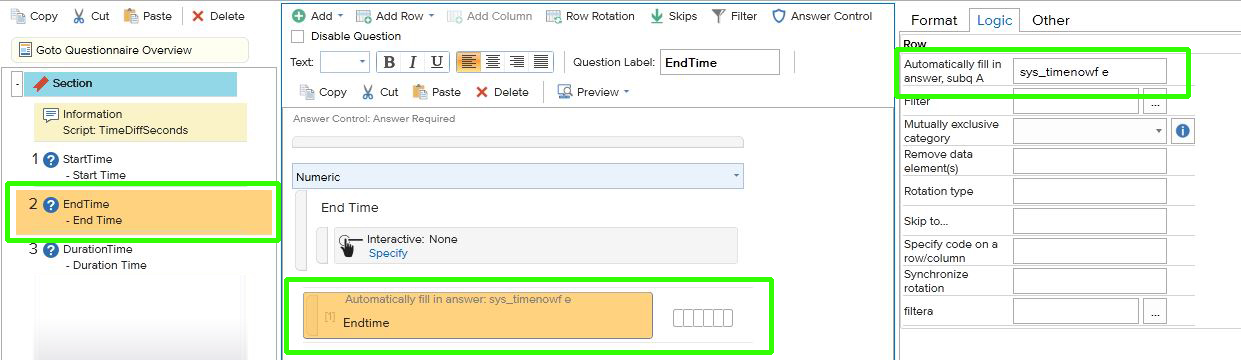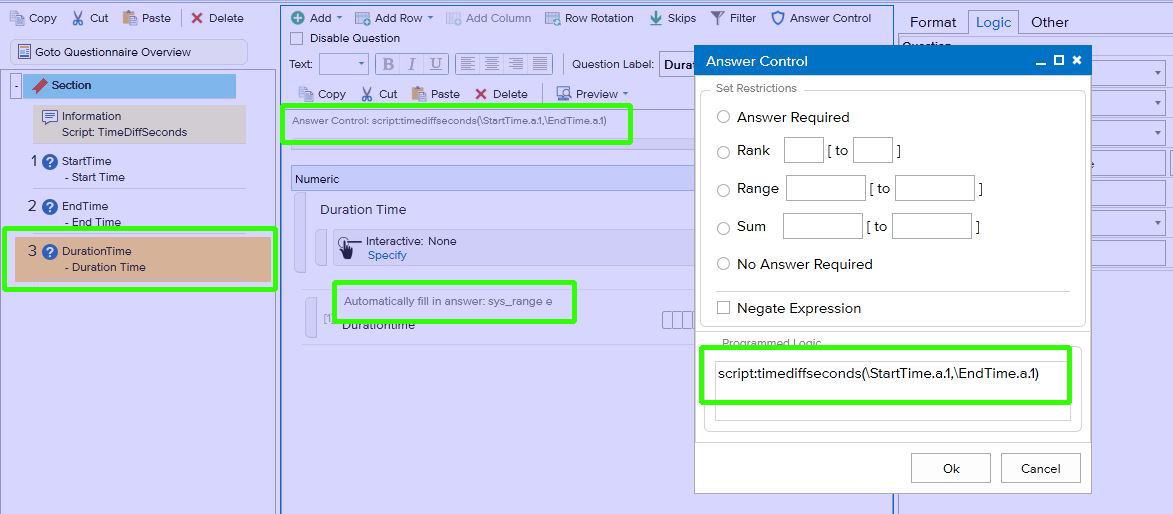# TimeDiffSeconds

Description:

script:timediffseconds(parameter1,parameter2)

Calculate the time difference between two paramters

Parameters:

This function has exactly 2 parameters

Parameter 1 = From time

Parameter 2 = End time

TimeStart and TimeEnd are numeric HHMMSS format

'TimeDiffSeconds = TimeEnd - TimeStart  (in Seconds)

Example:

script:timediffseconds(\StartTime.a.1,\EndTime.a.1)

StartTime

Use the function sys_timenowf to save the time in this numeric question (use e to show the answer, c if you want to hide the answer)EndTime

Use the function sys_timenowf again to save the time in this numeric question (use e to show the answer, c if you want to hide the answer)DurationTime

Use the script:timediffseconds to calculate the difference between StartTime and EndTime, use the sys_range to save the result in the answer option (use e to show the answer, c if you want to hide the answer)